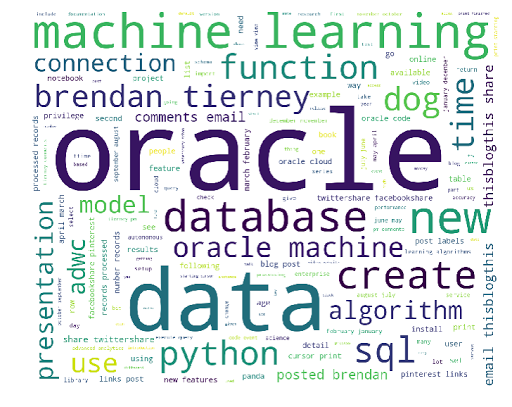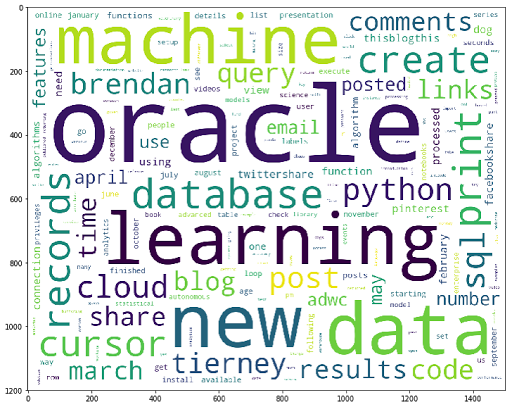In this section we will look at how to create a word cloud using Python. Yes there are lots of examples out there that show this, but none of them worked for me. This could be due to those examples using the older version of Python, libraries/packages no long exist, etc. There are lots of possible reasons. So I have to piece it together and the code given below is what I ended up with. Some steps could be skipped but this is what I ended up with.

Step 1 – Read in the data

In my example I wanted to create a word cloud for a website, so I picked my own blog for this exercise/example. The following code is used to read the website (a list of all packages used is given at the end).

```import nltk
from urllib.request import urlopen
from bs4 import BeautifulSoup

url = "http://www.oralytics.com/"
print(html)
```

The last line above, print(html), isn’t needed, but I used to to inspect what html was read from the webpage.

Step 2 – Extract just the Text from the webpage

The Beautiful soup library has some useful functions for processing html. There are many alternative ways of doing this processing but this is the approached that I liked.

The first step is to convert the downloaded html into BeautifulSoup format. When you view this converted data you will notices how everything is nicely laid out.

The second step is to remove some of the scripts from the code.

```soup = BeautifulSoup(html)
print(soup)

# kill all script and style elements
for script in soup(["script", "style"]):
script.extract() # rip it out

print(soup)
```

Step 3 – Extract plain text and remove whitespacing

The first line in the following extracts just the plain text and the remaining lines removes leading and trailing spaces, compacts multi-headlines and drops blank lines.

```text = soup.get_text()
print(text)

# break into lines and remove leading and trailing space on each
lines = (line.strip() for line in text.splitlines())
# break multi-headlines into a line each
chunks = (phrase.strip() for line in lines for phrase in line.split(" "))
# drop blank lines
text = '\n'.join(chunk for chunk in chunks if chunk)

print(text)
```

Step 4 – Remove stop words, tokenise and convert to lower case

As the heading says this code removes standard stop words for the English language, removes numbers and punctuation, tokenises the text into individual words, and then converts all words to lower case.

```#download and print the stop words for the English language
from nltk.corpus import stopwords
stop_words = set(stopwords.words('english'))
print(stop_words)

#tokenise the data set
from nltk.tokenize import sent_tokenize, word_tokenize
words = word_tokenize(text)
print(words)

# removes punctuation and numbers
wordsFiltered = [word.lower() for word in words if word.isalpha()]
print(wordsFiltered)

# remove stop words from tokenised data set
filtered_words = [word for word in wordsFiltered if word not in stopwords.words('english')]
print(filtered_words)
```

Step 5 – Create the Word Cloud

Finally we can create a word cloud backed on the finalised data set of tokenised words. Here we use the WordCloud library to create the word cloud and then the matplotlib library to display the image.

```from wordcloud import WordCloud
import matplotlib.pyplot as plt

wc = WordCloud(max_words=1000, margin=10, background_color='white',
scale=3, relative_scaling = 0.5, width=500, height=400,
random_state=1).generate(' '.join(filtered_words))

plt.figure(figsize=(20,10))
plt.imshow(wc)
plt.axis("off")
plt.show()
#wc.to_file("/wordcloud.png")
```

We get the following word cloud.Step 6 – Word Cloud based on frequency counts

Another alternative when using the WordCloud library is to generate a WordCloud based on the frequency counts. For this you need to build up a table containing two items. The first item is the distinct token and the second column contains the number of times that word/token appears in the text. The following code shows this code and the code to generate the word cloud based on this frequency count.

```from collections import Counter

# count frequencies
cnt = Counter()
for word in filtered_words:
cnt[word] += 1

print(cnt)

from wordcloud import WordCloud
import matplotlib.pyplot as plt

wc = WordCloud(max_words=1000, margin=10, background_color='white',
scale=3, relative_scaling = 0.5, width=500, height=400,
random_state=1).generate_from_frequencies(cnt)

plt.figure(figsize=(20,10))
plt.imshow(wc)
#plt.axis("off")
plt.show()
```

Now we get the following word cloud.When you examine these word cloud to can easily guess what the main contents of my blog is about. Machine Learning, Oracle SQL and coding.

What Python Packages did I use?

Here are the list of Python libraries that I used in the above code. You can use PIP3 to install these into your environment.

```nltk
url open
BeautifulSoup
wordcloud
Counter
```